# Copy and Paste Only Non-blank Cells

This post will guide you how to copy and paste only non-blank cells in Excel 2013/2016. How do I copy only non blank cells with VBA code in Excel. Or how to get non-blank cells from a range of cells with a formula in Excel.

This post will introduce four methods to copy and paste only non-blank cells in a selected range in Excel.

## Method1: Copy non-blank cells with Filter feature

If you want only copy non-blank cells in a range in Excel, you need to select the non-blank cells firstly, then press Ctrl +C keys to copy the selected cells. So how to only select all non-blank cells in the selected range in your worksheet. Just do the following steps:

#1 select the range of cells that you want to filter all non-blank cells.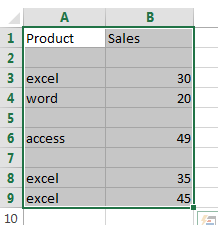#2 go to DATA tab, click Filter command under Sort & Filter group.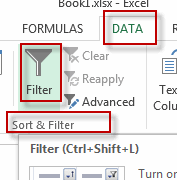#3 the drop-down button should appear in the first cell of your range. Click on this drop down button. de-select the (Blanks) option and then click on the OK button.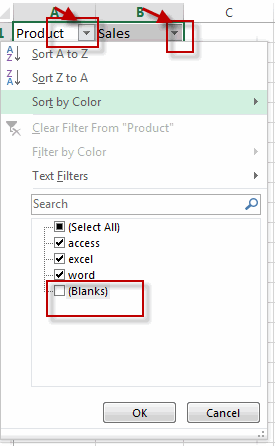#4 you will see that only non-blank cells are displayed in the selected range.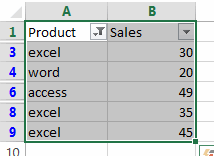#5 then you can select all non-blank cells in the current range and press Ctrl + C shortcuts in your keyboard, and then press Ctrl + V keys to paste the selected cells into a destination cell.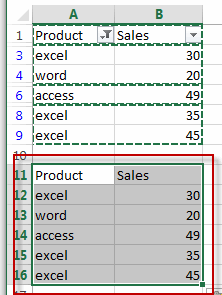## Method2: Copy non-blank Cells with Go To Special Feature

You can also use the Go To Special feature to select all non-blank cells in the selected range of cells. Do the following steps:

#1 select the range of cells that you want to filter all non-blank cells.#2 go to HOME tab, click Find & Select command under Editing group. And select Go To Special from the popup menu list. The Go To Special window will open.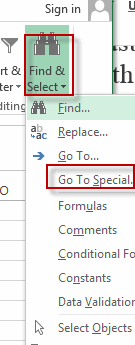#3 select Constants radio button in the Select section under Go To Special dialog. Then click OK button.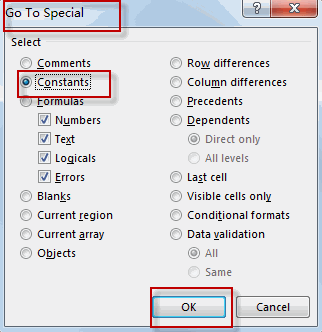#4 all non-blank cells in the selected range are highlighted.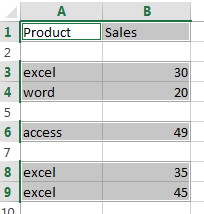#5 you can press Ctrl + C shortcuts to copy cells have been highlighted, and then press Ctrl + V keys to paste the selected cells into a destination cell.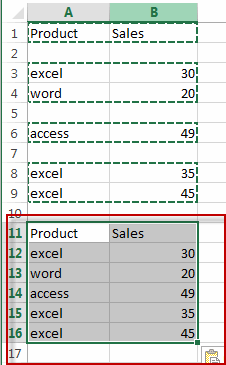## Method3: Copy non-blank Cells with VBA Macro

You can also use an Excel array formula based on the LOOKUP function, the CHOOSE function, the INDEX function, the SMALL function, the IF function, the ROW function and the ROWS function to extract all non-blank cells from a cell. Like this:

`=LOOKUP("xxxxx",CHOOSE({1,2},"",INDEX(A:A,SMALL(IF(\$A\$1:\$A\$9<>"",ROW(\$A\$1:\$A\$9)),ROWS(\$B\$1:B1)))))`

Type this formula into cell B1, and then press Ctrl +Shift +Enter shortcuts to change this formula as array formula. And then drag the AutoFill Handle from Cell B1 to B9.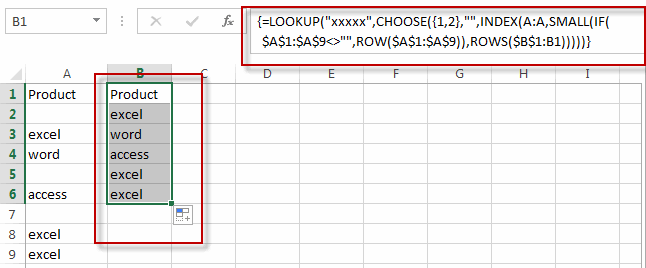All non-blank cells from the range A1:A9 are extracted in the range B1:B9.

## Method4: Copy non-blank Cells with Formula

You can also write an Excel VBA macro to copy and paste all non-blank cells, just do the following steps:

#1 select the range of cells that you want to filter all non-blank cells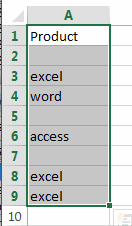#2 open your excel workbook and then click on “Visual Basic” command under DEVELOPER Tab, or just press “ALT+F11” shortcut.#3 then the “Visual Basic Editor” window will appear.

#4 click “Insert” ->”Module” to create a new module.#5 paste the below VBA code into the code window. Then clicking “Save” button.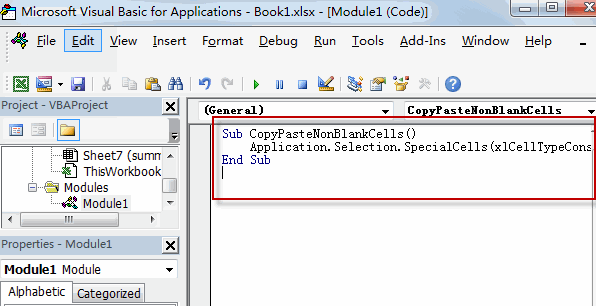```Sub CopyPasteNonBlankCells()
Application.Selection.SpecialCells(xlCellTypeConstants).Copy Destination:=Range("B1")
End Sub```

#6 back to the current worksheet, then run the above excel macro. Click Run button.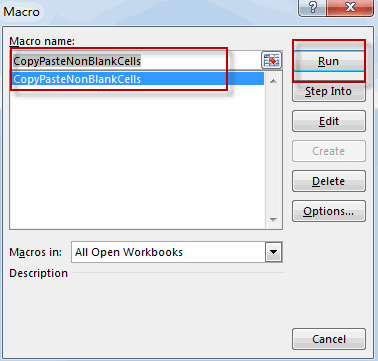#7 let’s see the result.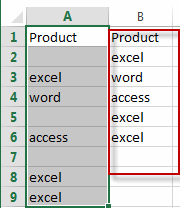### Related Functions

• Excel LOOKUP function
The Excel LOOKUP function will search a value in a vector or array.The LOOKUP function is a build-in function in Microsoft Excel and it is categorized as a Lookup and Reference Function.The syntax of the LOOKUP function is as below:= LOOKUP (lookup_value, lookup_vector, [result_vector])…
• Excel Choose Function
The Excel CHOOSE function returns a value from a list of values. The CHOOSE function is a build-in function in Microsoft Excel and it is categorized as a Lookup and Reference Function.The syntax of the CHOOSE function is as below:=CHOOSE (index_num, value1,[value2],…)…
• Excel INDEX function
The Excel INDEX function returns a value from a table based on the index (row number and column number)The INDEX function is a build-in function in Microsoft Excel and it is categorized as a Lookup and Reference Function.The syntax of the INDEX function is as below:= INDEX (array, row_num,[column_num])…
• Excel ROWS function
The Excel ROWS function returns the number of rows in a cell reference.The syntax of the ROWS function is as below:= ROWS(array)…
• Excel SMALL function
The Excel SMALL function returns the smallest numeric value from the numbers that you provided. Or returns the smallest value in the array.The syntax of the SMALL function is as below:=SMALL(array,nth) …
• Excel ROW function
The Excel ROW function returns the row number of a cell reference.The ROW function is a build-in function in Microsoft Excel and it is categorized as a Lookup and Reference Function.The syntax of the ROW function is as below:= ROW ([reference])….
• Excel IF function
The Excel IF function perform a logical test to return one value if the condition is TRUE and return another value if the condition is FALSE. The IF function is a build-in function in Microsoft Excel and it is categorized as a Logical Function.The syntax of the IF function is as below:= IF (condition, [true_value], [false_value])….
Related Posts

Find and Replace Multiple Values

This post will guide you how to find and replace multiple values at once with VBA macro or using formula  in Excel. How do I make multiple find and replace in Excel. Suppose that you have a few cells containing ...

VBA Macro For VLOOKUP From Another Sheet

In the previous post, you should know that how to fix or remove the #N/A error when using VLOOKUP formula to lookup value from another sheet. And this post will show you how to use VBA code to vlookup data ...

How To Insert Comments in Protected Worksheet in Excel

This post will show you how to allow comments in a protected worksheet in Excel. You can easily to insert comments into cells in a normal worksheet in Excel, but if want to insert a comment in a worksheet that ...

How To Convert Text to Upper Cases(Using VBA) in Excel

This post will show you how to switch from lower case to upper case in Excel. and I am going to show you two different ways of converting text to upper cases using formula or VBA macro in Excel 2013,Excel ...

How To Hide Every Other Row in Excel (Using VBA)

This post will show you how to hide alternate rows or columns in Excel or how to hide every third, fourth, fifth row or column in Excel. If you want to hide every other row in your current worksheet, how ...

Sidebar